# How to use excel sumif function?

In this article we will learn how to use Sumif function for count the number text contains cells on the bases of criteria in Excel.

While working in MS Excel when we prepare reports and dashboards, we come across situations wherein we need to sum multiple criteria based. Here we can use  excel SUMIF function.

Let’s understand how to use the SUMIF formula in different ways. Let’s take an excel file where each row of columns A & B contains text and any value respectively.

We want to sum all the numbers in column B corresponding with the text values in column A that meet certain criteria. These criteria have been listed in column F of each screenshot below. We will review one criteria a t time.

1. Text is “Excel”

The formula will be =SUMIF(A2:A6,”Excel”,B2:B6)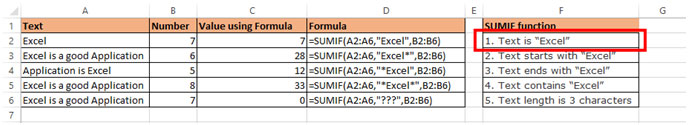This will sum all the values in column B where the corresponding cell in column A is equal to "Excel".

2. Text starts with “Excel”

=SUMIF(A2:A6,”Excel*”,B2:B6)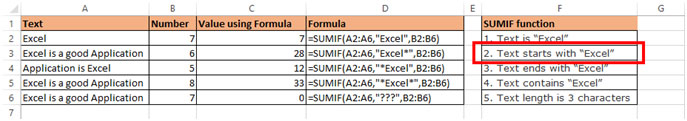This will sum all the values in column B where the corresponding cell in column A has text starting with "Excel".

3. Text ends with “Excel”

=SUMIF(A2:A6,”*Excel”,B2:B6)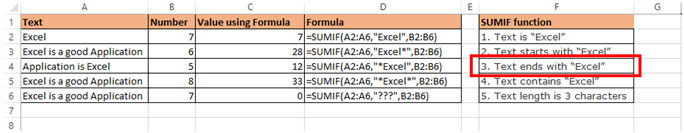This will sum all the values in column B where the corresponding cell in column A has text ending with "Excel".

4. Text contains “Excel”

=SUMIF(A2:A6,”*Excel*”,B2:B6)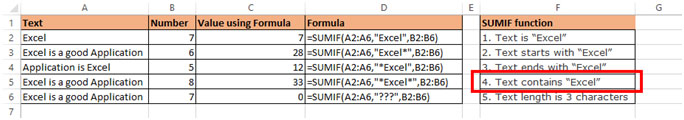This will sum all the values in column B where the corresponding cell in column A has text containing "Excel".

5. Text length is 3 characters

=SUMIF(A2:A6,”???”,B2:B6)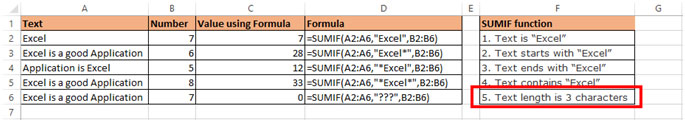This will sum all the values in column B where the corresponding cell in column A contains text of length 3 characters.

## Users are saying about us...

1.Hi!
I've tried to use this formula but it doesn't work on my Excel file, I use Excel 2003...

•Hey,

This formula will work in MS Excel-2003 as well.

2.Thanks! This information is invaluable.

3.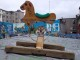Is there a way to do this with an "OR" function? So it searches for multiple terms?

Thanks!

•Hi Alex,

You can use SUMIFS function.

4.Thanks a lot ! for the great help 🙂

Terms and Conditions of use

The applications/code on this site are distributed as is and without warranties or liability. In no event shall the owner of the copyrights, or the authors of the applications/code be liable for any loss of profit, any problems or any damage resulting from the use or evaluation of the applications/code.